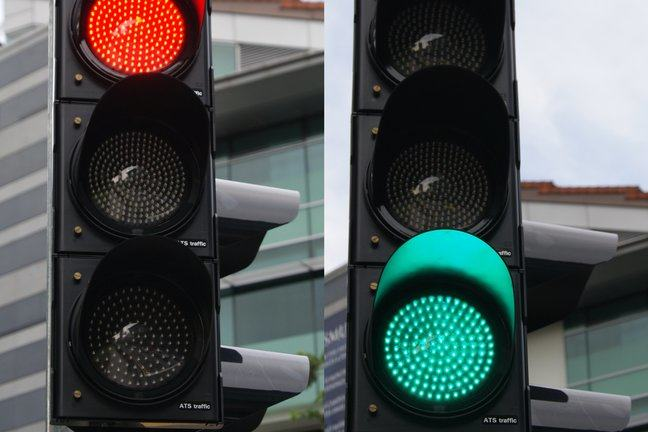## Want to keep learning?

This content is taken from the The University of Glasgow's online course, Functional Programming in Haskell: Supercharge Your Coding. Join the course to learn more.
4.11

## The University of GlasgowRed and Green Traffic Lights

# Using QuickCheck

Now let’s have a go at using QuickCheck for ourselves. We presume you have already watched the video about QuickCheck in the previous step.

We’ll run this session in GHCi — so we can type things interactively and see how we get on.

Let’s start by checking some properties of the abs function, that returns the absolute value of a number.

For positive numbers, the absolute value of a number is equal to itself, we could try to check this property.

import Test.QuickCheck
quickCheck ((\n->(abs(n) == n))::Int->Bool)


It’s helpful to put the types in here. We have created a lambda function that takes a single integer parameter then checks to see whether it is equal to its absolute value. When we run QuickCheck, we see this fails quickly — for negative numbers! This is a problem with our property specification, not with the abs function itself.

OK — so let’s write another check. We want to say that a value is always equal to its absolute value, or (0 - its absolute value)

quickCheck ((\n->(abs(n) == n) || (0-abs(n) ==n))::Int->Bool)


Great, it works! Now what else could we check? Maybe we could that the first element of a sorted list is its minimum, or that the last element is its maximum?

import Data.List
quickCheck ((\l->((minimum l) == (sort l)!!0))::[Int]->Bool)


Unfortunately this property fails for empty lists. So let’s refine our definition so it’s always true for empty lists.

quickCheck ((\l->(if l==[] then True else (minimum l) == (sort l)!!0))::[Int]->Bool)


Now it works — at least, it passes 100 tests. We can actually discover what these tests are — use verboseCheck instead of quickCheck.

Now we see why we had to specify the type for the list. This means QuickCheck generates random lists of the right type. Let’s try Char lists instead:

verboseCheck ((\l->(if l==[] then True else (minimum l) == (sort l)!!0))::[Char]->Bool)


This works fine, although most of the characters are unprintable.

I guess you might want to use QuickCheck for some of your own code — it’s very helpful for prototyping!

The QuViC company makes money using QuickCheck for various telecoms projects, see their website for details.